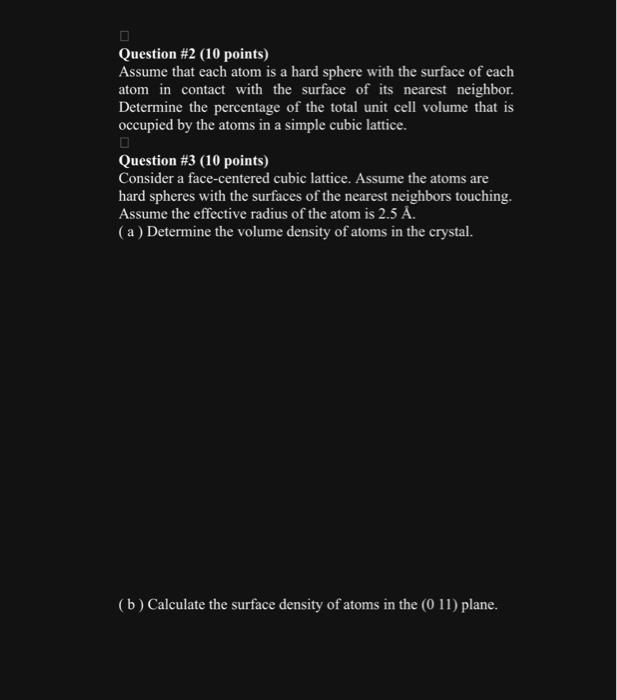Home / Expert Answers / Electrical Engineering / question-2-10-points-assume-that-each-atom-is-a-hard-sphere-with-the-surface-of-each-atom-in-con-pa318

# (Solved): Question #2 (10 points) Assume that each atom is a hard sphere with the surface of each atom in con ...Question #2 (10 points) Assume that each atom is a hard sphere with the surface of each atom in contact with the surface of its nearest neighbor. Determine the percentage of the total unit cell volume that is occupied by the atoms in a simple cubic lattice. Question #3 (10 points) Consider a face-centered cubic lattice. Assume the atoms are hard spheres with the surfaces of the nearest neighbors touching. Assume the effective radius of the atom is $$2.5 \mathrm{~A}$$. (a) Determine the volume density of atoms in the crystal. (b) Calculate the surface density of atoms in the $$(0$$ 11) plane.

We have an Answer from Expert